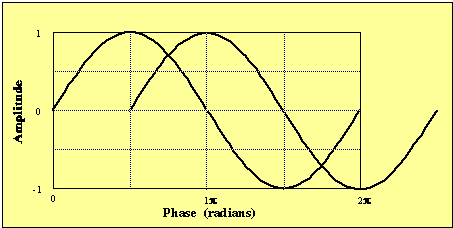# Superposition of waves - constructive or destructive interfernce?

Hi Guys,

I wonder if someone could help check something for me in order to make sure that I'm not making a stupid mistake with this problem as I've been marked wrong on an undergraduate paper, but I'm almost certain that I'm right. I don't quite have enough courage in my convictions to approach the tutor yet, hence why I'm posting this thread.

## Homework Statement

The question relates to the superposition of waves and destructive and constructive interference in various types of microscopes, and the question as stated is as follows:

"The two light waves below are shifted one quarter out of phase, draw the resultant wave if these are combined"

The paper shows two waves of equal amplitude, frequency, and wavelength, propagated in the same direction, a quarter of a wavelength out of phase.

The feedback I received was that the resultant amplitude should be smaller than the original, as destructive interference occurs, while I drew a wave which was amplified showing constructive interference.

## Homework Equations

The one i'm using is:

Resultant amplitude = 2 x Amplitude (cos [phase shift in radians/2])

I hope that's correct.

## The Attempt at a Solution

I assume I'm correct in stating there are 360 degrees in a wave cycle, so in radians that would be 2 times pi, or approximately 6.283.

If the waves are 1/4 out of phase, the phase shift in radians is 2pi/4 (or pi/2), and so therefore approximately 1.57. Dividing this by 2 we get 0.785, and cos of this (in radians) is 0.707. If we take the amplitude of the original waves as 1, then the resultant amplitude is (2 x 1) x 0.707 = 1.414. The resultant amplitude is therefore larger than the original amplitude.

The formula seems to be correct since at half a wavelength out of phase, which is where I know the resultant amplitude is zero, the answer comes out at zero: (2x1) x cos (pi/2) = 2 x 0 = 0

And when maximum constructive interference occurs, i.e. the waves are in phase, (2x1) x cos (0/2) = (2x1) x 1 = 2

Assuming the equation is correct, you would have to shift a third out of phase before the amplitude matches the original amplitude, and then between a third and half a wavelength out of phase, the interference is destructive down to zero:

(2x1) x cos(2.094/2) = 2 x 0.5 = 1

So anyway, can anyone spot a mistake?

Thanks for any help!

CWatters
Homework Helper
Gold Member
Can you post the drawing? If they are definitely 1/4 wavelength out of phase like this?You also mention "various types of microscopes". Is it possible one ray becomes inverted before the interference occurs?

Thanks for replying but the diagram is actually quite a rough one so it would be of little use, even if I could post it. The question is exactly as I stated in the OP, i.e.

"The two light waves below are shifted one quarter out of phase, draw the resultant wave if these are combined"

and the waves are identical except for the shift you have shown above.

The inaccurate original diagram, the lack of graph paper for the answer, and the feedback stating simply that the amplitude is less than the original (and therefore destructive interference), leads me to believe that they were not looking for a precise representation of the resultant wave, but rather a demonstration that we understood the principle at work, but I disagree with their assertion that the interference is overall destructive.

Oh and mentioning microscopy was not really relevant to the question, I was just setting the context of the class where the question was asked. It's not a physics class, more medical related (and for that reason I have some reason to suspect that the tutors are not experts in this area ;) - although neither am I!).

CWatters
•